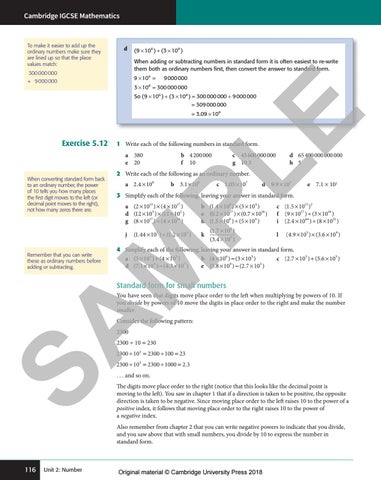# Preview Cambridge IGCSE Mathematics Core and Extended Coursebook Second EditionCambridge IGCSE Mathematics

To make it easier to add up the ordinary numbers make sure they are lined up so that the place values match:

d

( 9 × 106 ) + (3 × 108 ) When adding or subtracting numbers in standard form it is often easiest to re-write them both as ordinary numbers first, then convert the answer to standard form.

300 000 000

9 × 106 =

+ 9 000 000

9 000 000

3 × 10 = 300 000 000 8

So ( 9 × 106 ) + (3 × 108 ) = 300 000 000 + 9 000 000 = 309 000 000

Exercise 5.12

E

= 3.09 × 108

1 Write each of the following numbers in standard form. a 380 b 4 200 000 e 20 f 10

2 Write each of the following as an ordinary number. a 2.4 × 106

b  3.1 × 108

c  1.05 × 107

d  9.9 × 103

e 7.1 × 101

3 Simplify each of the following, leaving your answer in standard form.

b (1.4 × 108 ) × (3 × 104 ) c (1.5 × 1013 )2 a (2 × 1013 ) × (4 × 1017 ) 5 2 17 16 d (12 × 10 ) × (11 × 10 ) e (0.2 × 10 ) × (0.7 × 10 ) f (9 × 1017 ) ÷ (3 × 1016 ) g (8 × 1017 ) ÷ (4 × 1016 ) h (1.5 × 108 ) ÷ (5 × 10 4 ) i (2.4 × 1064 ) ÷ (8 × 1021 ) j (1.44 × 107 ) ÷ (1.2 × 104 )

k

(1.7 × 108 ) (3.4 × 105 )

l

(4.9 × 105 ) × (3.6 × 109 )

4 Simplify each of the following, leaving your answer in standard form. a (3 × 104 ) + (4 × 103 ) b (4 × 106 ) − (3 × 105 ) c (2.7 × 103 ) + (5.6 × 105 ) 9 7 9 3 d (7.1 × 10 ) − (4.3 × 10 ) e (5.8 × 10 ) − (2.7 × 10 )

SA M

Remember that you can write these as ordinary numbers before adding or subtracting.

d 65 400 000 000 000 h 5

PL

When converting standard form back to an ordinary number, the power of 10 tells you how many places the first digit moves to the left (or decimal point moves to the right), not how many zeros there are.

c 45 600 000 000 g 10.3

Standard form for small numbers You have seen that digits move place order to the left when multiplying by powers of 10. If you divide by powers of 10 move the digits in place order to the right and make the number smaller. Consider the following pattern: 2300

2300 ÷ 10 = 230

2300 ÷ 102 = 2300 ÷ 100 = 23

2300 ÷ 103 = 2300 ÷ 1000 = 2.3 . . . and so on.

The digits move place order to the right (notice that this looks like the decimal point is moving to the left). You saw in chapter 1 that if a direction is taken to be positive, the opposite direction is taken to be negative. Since moving place order to the left raises 10 to the power of a positive index, it follows that moving place order to the right raises 10 to the power of a negative index. Also remember from chapter 2 that you can write negative powers to indicate that you divide, and you saw above that with small numbers, you divide by 10 to express the number in standard form.

116

Unit 2: Number

9781108437189c05_p101-122.indd 116

Original material © Cambridge University Press 2018 15-Jan-18 10:26:16 PM